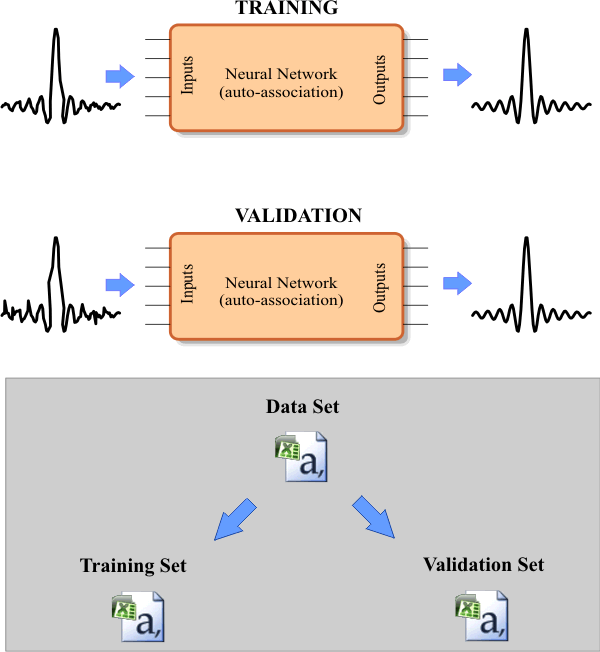# The sinc function (single data set)

 Problem 1 Create a New Project called AssoSinc to build an appropriate data set for learning of the sinc signal z = sinc(x) using an ANN for auto-association as shown in the figure (BuildDateSet.lab file). Use an appropriate number of cases and inputs. The input should be contaminated with 10 % of noise.Problem 2 Add a file called Split.lab to split the data set to create the training set and the validation set.

 Problem 3 Edit the Train.lab file to design and train an ANN for z = sinc(x). Design the type of training and the training parameters to get the best results.

 Problem 4 Edit the CheckTraining.lab file to check the training: (a) Compute the mean squared error for the ANN using the training set. (b) Plot the error for each training case. (c) Save the plot as a vector image (checkTraining.pdf and checkTraining.emf)

 Problem 5 Edit the Validation.lab file to perform the validation of the ANN. (a) Compute the mean squared error for the ANN using the validation set. (b) Plot the error for each validation case. (c) Save the plot as a vector image (validation.pdf and validation.emf)

 Problem 6 Generate a report in Microsoft Word. Write some conclusions in the report focusing on the problems that were faced during the simulation and how these problems were or could be solved.